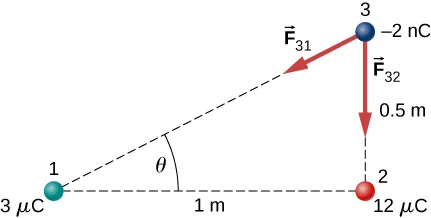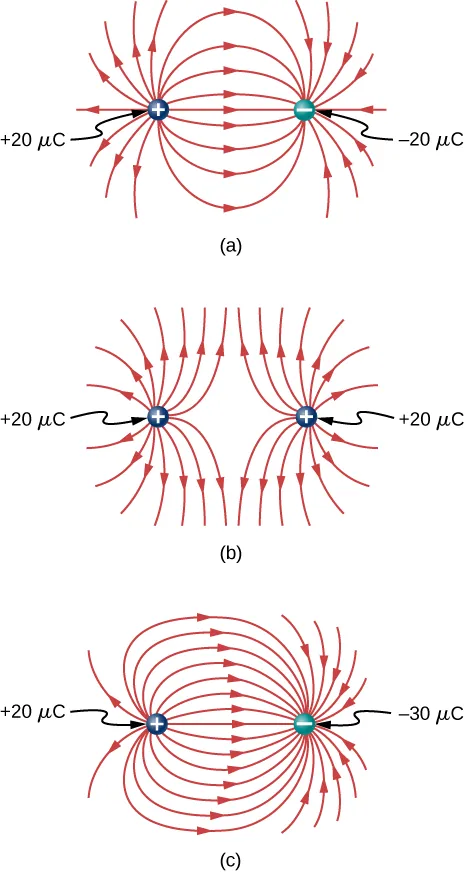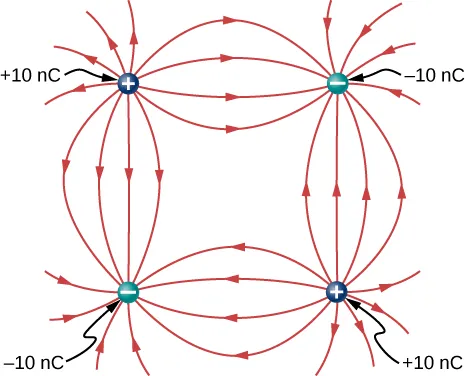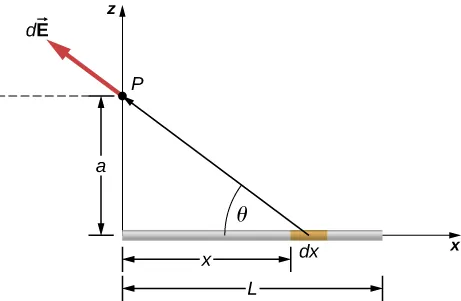University Physics Volume 2

# Chapter 5

5.1

The force would point outward.

5.2

The net force would point $58°58°$ below the −x-axis.

5.3

$E → = 1 4 π ε 0 q r 2 r ^ E → = 1 4 π ε 0 q r 2 r ^$

5.4

We will no longer be able to take advantage of symmetry. Instead, we will need to calculate each of the two components of the electric field with their own integral.

5.5

The point charge would be $Q=σabQ=σab$ where a and b are the sides of the rectangle but otherwise identical.

5.6

The electric field would be zero in between, and have magnitude $σε0σε0$ everywhere else.

### Conceptual Questions

1.

There are mostly equal numbers of positive and negative charges present, making the object electrically neutral.

3.

a. no; b. yes

5.

Take an object with a known charge, either positive or negative, and bring it close to the rod. If the known charged object is positive and it is repelled from the rod, the rod is charged positive. If the positively charged object is attracted to the rod, the rod is negatively charged.

7.

No, the dust is attracted to both because the dust particle molecules become polarized in the direction of the silk.

9.

Yes, polarization charge is induced on the conductor so that the positive charge is nearest the charged rod, causing an attractive force.

11.

Charging by conduction is charging by contact where charge is transferred to the object. Charging by induction first involves producing a polarization charge in the object and then connecting a wire to ground to allow some of the charge to leave the object, leaving the object charged.

13.

This is so that any excess charge is transferred to the ground, keeping the gasoline receptacles neutral. If there is excess charge on the gasoline receptacle, a spark could ignite it.

15.

The dryer charges the clothes. If they are damp, the presence of water molecules suppresses the charge.

17.

There are only two types of charge, attractive and repulsive. If you bring a charged object near the quartz, only one of these two effects will happen, proving there is not a third kind of charge.

19.

a. No, since a polarization charge is induced. b. Yes, since the polarization charge would produce only an attractive force.

21.

The force holding the nucleus together must be greater than the electrostatic repulsive force on the protons.

23.

Either sign of the test charge could be used, but the convention is to use a positive test charge.

25.

The charges are of the same sign.

27.

At infinity, we would expect the field to go to zero, but because the sheet is infinite in extent, this is not the case. Everywhere you are, you see an infinite plane in all directions.

29.

The infinite charged plate would have $E=σ2ε0E=σ2ε0$ everywhere. The field would point toward the plate if it were negatively charged and point away from the plate if it were positively charged. The electric field of the parallel plates would be zero between them if they had the same charge, and E would be $E=σε0E=σε0$ everywhere else. If the charges were opposite, the situation is reversed, zero outside the plates and $E=σε0E=σε0$ between them.

31.

yes; no

33.

At the surface of Earth, the gravitational field is always directed in toward Earth’s center. An electric field could move a charged particle in a different direction than toward the center of Earth. This would indicate an electric field is present.

35.

10

### Problems

37.

a. $2.00×10−9C(11.602×10−19e/C)=1.248×1010electrons2.00×10−9C(11.602×10−19e/C)=1.248×1010electrons$;
b. $0.500×10−6C(11.602×10−19e/C)=3.121×1012electrons0.500×10−6C(11.602×10−19e/C)=3.121×1012electrons$

39.

$3.750 × 10 21 e 6.242 × 10 18 e / C = –600.8 C 3.750 × 10 21 e 6.242 × 10 18 e / C = –600.8 C$

41.

a. $2.0×10−9C(6.242×1018e/C)=1.248×1010e2.0×10−9C(6.242×1018e/C)=1.248×1010e$;

b. $9.109×10−31kg(1.248×1010e)=1.137×10−20kg,9.109×10−31kg(1.248×1010e)=1.137×10−20kg,$ $1.137×10−20kg2.5×10−3kg=4.548×10−18or4.545×10−16%1.137×10−20kg2.5×10−3kg=4.548×10−18or4.545×10−16%$

43.

$5.00×10−9C(6.242×1018e/C)=3.121×1010e5.00×10−9C(6.242×1018e/C)=3.121×1010e$;
$3.121×1010e+1.0000×1012e=1.0312×1012e3.121×1010e+1.0000×1012e=1.0312×1012e$

45.

atomic mass of copper atom times $1u=1.055×10−25kg1u=1.055×10−25kg$;
number of copper atoms $=4.739×1023atoms=4.739×1023atoms$;
number of electrons equals 29 times number of atoms or $1.374×1025electrons1.374×1025electrons$; $2.00×10−6C(6.242×1018e/C)1.374×1025e=9.083×10−13or9.083×10−11%2.00×10−6C(6.242×1018e/C)1.374×1025e=9.083×10−13or9.083×10−11%$

47.

$244.00u(1.66×10−27kg/u)=4.050×10−25kg244.00u(1.66×10−27kg/u)=4.050×10−25kg$;
$4.00kg4.050×10−25kg=9.877×1024atoms9.877×1024(94)=9.284×1026protons4.00kg4.050×10−25kg=9.877×1024atoms9.877×1024(94)=9.284×1026protons$;
$9.284×1026(1.602×10−19C/p)=1.487×108C9.284×1026(1.602×10−19C/p)=1.487×108C$

49.

a. charge 1 is $3μC3μC$; charge 2 is $12μC12μC$, $F31=2.16×10−4NF31=2.16×10−4N$ to the left,
$F32=8.63×10−4NF32=8.63×10−4N$ to the right,
$Fnet=6.47×10−4NFnet=6.47×10−4N$ to the right;
b. $F31=2.16×10−4NF31=2.16×10−4N$ to the right,
$F32=9.59×10−5NF32=9.59×10−5N$ to the right,
$Fnet=3.12×10−4NFnet=3.12×10−4N$ to the right,;
c. $F→31x=−3.86×10−5Ni^F→31x=−3.86×10−5Ni^$,
$F→31y=−1.73×10−5Nj^F→31y=−1.73×10−5Nj^$,
$F→32y=−8.63×10−4Nj^F→32y=−8.63×10−4Nj^$
$F→net=−3.86×10−5Ni^−8.82×10−4Nj^F→net=−3.86×10−5Ni^−8.82×10−4Nj^$

51.

$F = 230.7 N F = 230.7 N$

53.

$F = 53.94 N F = 53.94 N$

55.

The tension is $T=0.049NT=0.049N$. The horizontal component of the tension is $0.0043N0.0043N$
$d=0.088m,q=6.1×10−8Cd=0.088m,q=6.1×10−8C$.
The charges can be positive or negative, but both have to be the same sign.

57.

Let the charge on one of the spheres be nQ, where n is a fraction between 0 and 1. In the numerator of Coulomb’s law, the term involving the charges is $nQ(1−n)Q.nQ(1−n)Q.$ This is equal to $(n−n2)Q2(n−n2)Q2$. Finding the maximum of this term gives $1−2n=0⇒n=121−2n=0⇒n=12$

59.

Define right to be the positive direction and hence left is the negative direction, then $F=−0.05NF=−0.05N$

61.

The particles form triangle of sides 13, 13, and 24 cm. The x-components cancel, whereas there is a contribution to the y-component from both charges 24 cm apart. The y-axis passing through the third charge bisects the 24-cm line, creating two right triangles of sides 5, 12, and 13 cm.
$Fy=2.56NFy=2.56N$ in the negative y-direction since the force is attractive. The net force from both charges is $F→net=−5.12Nj^F→net=−5.12Nj^$.

63.

The diagonal is $2a2a$ and the components of the force due to the diagonal charge has a factor $cosθ=12cosθ=12$;
$F→net=[kq2a2+kq22a212]i^−[kq2a2+kq22a212]j^F→net=[kq2a2+kq22a212]i^−[kq2a2+kq22a212]j^$

65.

a. $E=2.0×102NCE=2.0×102NC$ up;
b. $F=2.0×10−6NF=2.0×10−6N$ down

67.

a. $E=2.88×1011N/CE=2.88×1011N/C$;
b. $E=1.44×1011N/CE=1.44×1011N/C$;
c. $F=4.61×10−8NF=4.61×10−8N$ on alpha particle;
$F=4.61×10−8NF=4.61×10−8N$ on electron

69.

$E = ( −2.0 i ^ + 3.0 j ^ ) N E = ( −2.0 i ^ + 3.0 j ^ ) N$

71.

$F=3.204×10−14NF=3.204×10−14N$,
$a=3.517×1016m/s2a=3.517×1016m/s2$

73.

$q = 2.78 × 10 −9 C q = 2.78 × 10 −9 C$

75.

a. $E=1.15×1012N/CE=1.15×1012N/C$;
b. $F=1.47×10−6NF=1.47×10−6N$

77.

If the $q2q2$ is to the right of $q1,q1,$ the electric field vector from both charges point to the right. a. $E=2.70×106N/CE=2.70×106N/C$;
b. $F=54.0NF=54.0N$

79.

There is $45°45°$ right triangle geometry. The x-components of the electric field at $y=3my=3m$ cancel. The y-components give $E(y=3m)=2.83×103N/CE(y=3m)=2.83×103N/C$.
At the origin we have a a negative charge of magnitide
$q=−2.83×10−6Cq=−2.83×10−6C$.

81.

$E → ( z ) = 3.6 × 10 4 N / C k ^ E → ( z ) = 3.6 × 10 4 N / C k ^$

83.

$d E = 1 4 π ε 0 λ d x ( x + a ) 2 , E = λ 4 π ε 0 [ 1 l + a − 1 a ] d E = 1 4 π ε 0 λ d x ( x + a ) 2 , E = λ 4 π ε 0 [ 1 l + a − 1 a ]$

85.

$σ = 0.02 C / m 2 E = 2.26 × 10 9 N / C σ = 0.02 C / m 2 E = 2.26 × 10 9 N / C$

87.

At $P1P1$: $E→(y)=14πε0λLyy2+L24j^⇒14πε0qa2(a2)2+L24j^=1πε0qaa2+L2j^E→(y)=14πε0λLyy2+L24j^⇒14πε0qa2(a2)2+L24j^=1πε0qaa2+L2j^$
At $P2:P2:$ Put the origin at the end of L.
$dE=14πε0λdx(x+a)2,E→=−q4πε0l[1l+a−1a]i^dE=14πε0λdx(x+a)2,E→=−q4πε0l[1l+a−1a]i^$

89.

a. $E→(r→)=14πε02λyai^+14πε02λxbj^E→(r→)=14πε02λyai^+14πε02λxbj^$; b. $14πε02(λx+λy)ck^14πε02(λx+λy)ck^$

91.

a. $F→=3.2×10−17Ni^F→=3.2×10−17Ni^$,
$a→=1.92×1010m/s2i^a→=1.92×1010m/s2i^$;
b. $F→=−3.2×10−17Ni^F→=−3.2×10−17Ni^$,
$a→=−3.51×1013m/s2i^a→=−3.51×1013m/s2i^$

93.

$m=6.5×10−11kgm=6.5×10−11kg$,
$E=1.6×107N/CE=1.6×107N/C$

95.

$E=1.70×106N/CE=1.70×106N/C$,
$F=1.53×10−3NTcosθ=mgTsinθ=qEF=1.53×10−3NTcosθ=mgTsinθ=qE$,
$tanθ=0.62⇒θ=32.0°tanθ=0.62⇒θ=32.0°$,
This is independent of the length of the string.

97.

circular arc $dEx(−i^)=14πε0λdsr2cosθ(−i^)dEx(−i^)=14πε0λdsr2cosθ(−i^)$,
$E→x=λ4πε0r(−i^)E→x=λ4πε0r(−i^)$,
$dEy(−i^)=14πε0λdsr2sinθ(−j^)dEy(−i^)=14πε0λdsr2sinθ(−j^)$,
$E→y=λ4πε0r(−j^)E→y=λ4πε0r(−j^)$;
y-axis: $E→x=λ4πε0r(−i^)E→x=λ4πε0r(−i^)$;
x-axis: $E→y=λ4πε0r(−j^)E→y=λ4πε0r(−j^)$,
$E→=λ2πε0r(−i^)+λ2πε0r(−j^)E→=λ2πε0r(−i^)+λ2πε0r(−j^)$

99.

a. $W=12m(v2−v02)W=12m(v2−v02)$, $Qq4πε0(1r−1r0)=12m(v2−v02)⇒r0−r=4πε0Qq12rr0m(v2−v02)Qq4πε0(1r−1r0)=12m(v2−v02)⇒r0−r=4πε0Qq12rr0m(v2−v02)$; b. $r0−rr0−r$ is negative; therefore, $v0>vv0>v$, $r→∞,andv→0:Qq4πε0(−1r0)=−12mv02⇒v0=Qq2πε0mr0r→∞,andv→0:Qq4πε0(−1r0)=−12mv02⇒v0=Qq2πε0mr0$

101.103.105.

$Ex=0,Ex=0,$
$Ey=14πε0[2q(x2+a2)a(x2+a2)]Ey=14πε0[2q(x2+a2)a(x2+a2)]$
$⇒x≫a⇒12πε0qax3⇒x≫a⇒12πε0qax3$,
$Ey=q4πε0[2ya+2ya(y−a)2(y+a)2]Ey=q4πε0[2ya+2ya(y−a)2(y+a)2]$
$⇒y≫a⇒1πε0qay3⇒y≫a⇒1πε0qay3$

107.

The net dipole moment of the molecule is the vector sum of the individual dipole moments between the two O-H. The separation O-H is 0.9578 angstroms:
$p→=1.889×10−29Cmi^p→=1.889×10−29Cmi^$

109.

$F→net=[−8.99×1093.0×10−6(5.0×10−6)(3.0m)2−8.99×1099.0×10−6(5.0×10−6)(3.0m)2]i^F→net=[−8.99×1093.0×10−6(5.0×10−6)(3.0m)2−8.99×1099.0×10−6(5.0×10−6)(3.0m)2]i^$,
$−8.99×1096.0×10−6(5.0×10−6)(3.0m)2j^=−0.06Ni^−0.03Nj^−8.99×1096.0×10−6(5.0×10−6)(3.0m)2j^=−0.06Ni^−0.03Nj^$

111.

Charges Q and q form a right triangle of sides 1 m and $3+3m.3+3m.$ Charges 2Q and q form a right triangle of sides 1 m and $3m.3m.$
$Fx=0.049N,Fx=0.049N,$
$Fy=0.093NFy=0.093N$,
$F→net=0.036Ni^+0.09Nj^F→net=0.036Ni^+0.09Nj^$

113.

$W = 0.054 J W = 0.054 J$

115.

a. $E→=14πε0(q(2a)2−qa2)i^E→=14πε0(q(2a)2−qa2)i^$; b. $E→=34πε0qa2(−j^)E→=34πε0qa2(−j^)$; c. $E→=2πε0qa212(−j^)E→=2πε0qa212(−j^)$

117.

$E → net = E → 1 + E → 2 + E → 3 + E → 4 = ( 4.65 i ^ + 1.44 j ^ ) × 10 7 N/C E → net = E → 1 + E → 2 + E → 3 + E → 4 = ( 4.65 i ^ + 1.44 j ^ ) × 10 7 N/C$

119.

$F=qE0(1+x/a)W=12m(v2−v02)F=qE0(1+x/a)W=12m(v2−v02)$,
$12mv2=qE0(15a2)J12mv2=qE0(15a2)J$

121.

Electric field of wire at x: $E→(x)=14πε02λyxi^E→(x)=14πε02λyxi^$,
$dF=λyλx2πε0(lnb−lna)dF=λyλx2πε0(lnb−lna)$

123.$dEx=14πε0λdx(x2+a2)xx2+a2dEx=14πε0λdx(x2+a2)xx2+a2$,
$E→x=λ4πε0[1L2+a2−1a]i^E→x=λ4πε0[1L2+a2−1a]i^$,
$dEz=14πε0λdx(x2+a2)ax2+a2dEz=14πε0λdx(x2+a2)ax2+a2$,
$E→z=λ4πε0aLL2+a2k^E→z=λ4πε0aLL2+a2k^$,
Substituting z for a, we have:
$E→(z)=λ4πε0[1L2+z2−1z]i^+λ4πε0zLL2+z2k^E→(z)=λ4πε0[1L2+z2−1z]i^+λ4πε0zLL2+z2k^$

125.

There is a net force only in the y-direction. Let $θθ$ be the angle the vector from dx to q makes with the x-axis. The components along the x-axis cancel due to symmetry, leaving the y-component of the force.
$dFy=14πε0aqλdx(x2+a2)3/2dFy=14πε0aqλdx(x2+a2)3/2$,
$Fy=12πε0qλa[l/2((l/2)2+a2)1/2]Fy=12πε0qλa[l/2((l/2)2+a2)1/2]$

Order a print copy

As an Amazon Associate we earn from qualifying purchases.